Java 中九种 Map 的遍历方式，你一般用的是哪种呢？• 累计撰写 146 篇文章
• 累计收到 14 条评论

# Java 中九种 Map 的遍历方式，你一般用的是哪种呢？2022-12-13 / 0 评论 / 58 阅读 / 正在检测是否收录...

### 1、通过 for 和 map.entrySet() 来遍历

第一种方式是采用 for 和 Map.Entry 的形式来遍历，通过遍历 map.entrySet() 获取每个 entry 的 key 和 value，代码如下。这种方式一般也是阿粉使用的比较多的一种方式，没有什么花里胡哨的用法，就是很朴素的获取 map 的 key 和 value。

``````public static void testMap1(Map<Integer, Integer> map) {
long sum = 0;
for (Map.Entry<Integer, Integer> entry : map.entrySet()) {
sum += entry.getKey() + entry.getValue();
}
System.out.println(sum);
}``````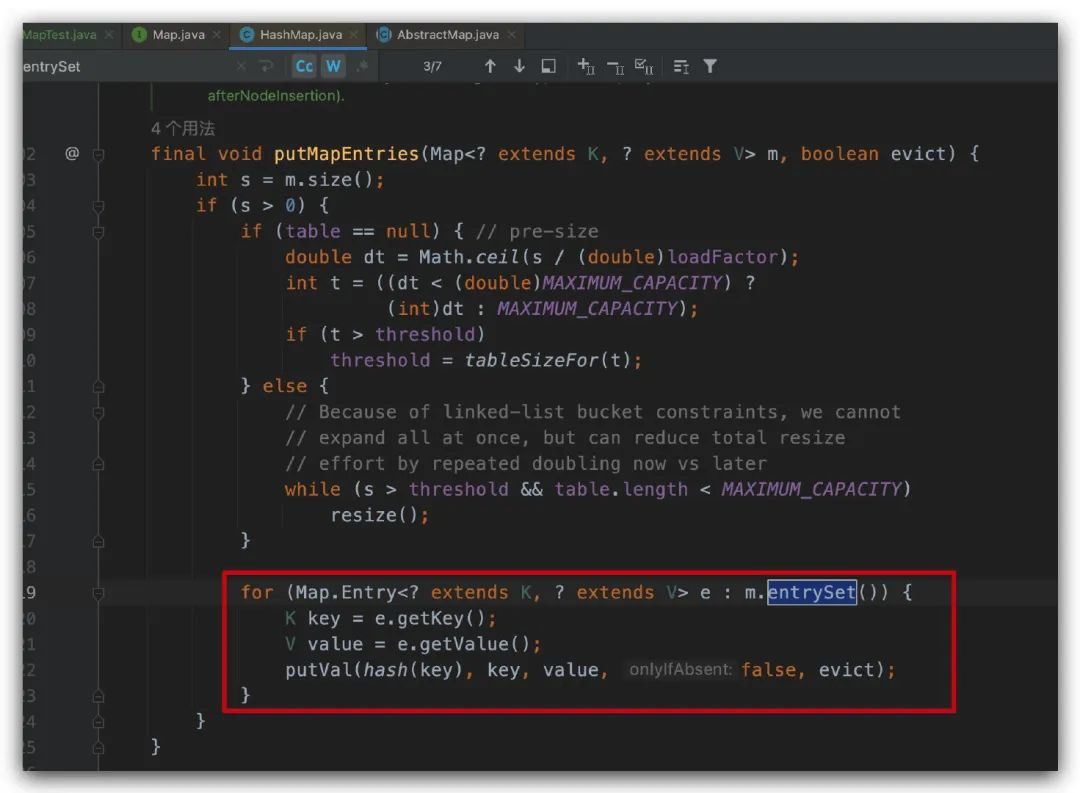putMapEntries 方法在我们调用 putAll 方法的时候会用到。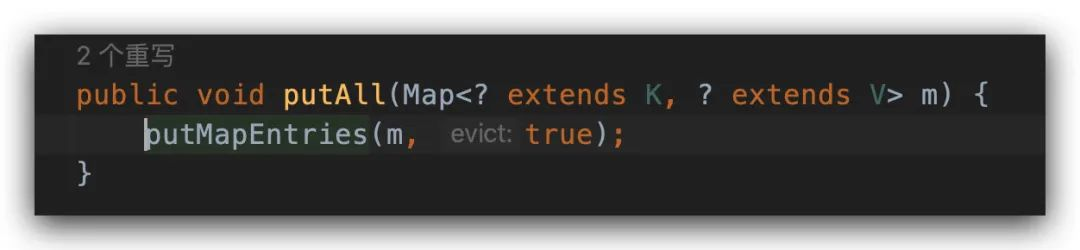### 2、通过 for， Iterator 和 map.entrySet() 来遍历

我们第一个方法是直接通过 for 和 entrySet() 来遍历的，这次我们使用 entrySet() 的迭代器来遍历，代码如下。

``````public static void testMap2(Map<Integer, Integer> map) {
long sum = 0;
for (Iterator<Map.Entry<Integer, Integer>> entries = map.entrySet().iterator(); entries.hasNext(); ) {
Map.Entry<Integer, Integer> entry = entries.next();
sum += entry.getKey() + entry.getValue();
}
System.out.println(sum);
}``````

### 3、通过 while，Iterator 和 map.entrySet() 来遍历

`````` public static void testMap3(Map<Integer, Integer> map) {
Iterator<Map.Entry<Integer, Integer>> it = map.entrySet().iterator();
long sum = 0;
while (it.hasNext()) {
Map.Entry<Integer, Integer> entry = it.next();
sum += entry.getKey() + entry.getValue();
}
System.out.println(sum);
}``````

### 4、通过 for 和 map.keySet() 来遍历

``````public static void testMap4(Map<Integer, Integer> map) {
long sum = 0;
for (Integer key : map.keySet()) {
sum += key + map.get(key);
}
System.out.println(sum);
}``````

### 5、通过 for，Iterator 和 map.keySet() 来遍历

``````public static void testMap5(Map<Integer, Integer> map) {
long sum = 0;
for (Iterator<Integer> key = map.keySet().iterator(); key.hasNext(); ) {
Integer k = key.next();
sum += k + map.get(k);
}
System.out.println(sum);
}``````

### 6、通过 while，Iterator 和 map.keySet() 来遍历

``````public static void testMap6(Map<Integer, Integer> map) {
Iterator<Integer> it = map.keySet().iterator();
long sum = 0;
while (it.hasNext()) {
Integer key = it.next();
sum += key + map.get(key);
}
System.out.println(sum);
}``````

### 7、通过 map.forEach() 来遍历

JDK 中的 forEach 方法，使用率也挺高的。

``````public static void testMap7(Map<Integer, Integer> map) {
final long[] sum = {0};
map.forEach((key, value) -> {
sum += key + value;
});
System.out.println(sum);
}``````### 8、Stream 遍历

``````public static void testMap8(Map<Integer, Integer> map) {
long sum = map.entrySet().stream().mapToLong(e -> e.getKey() + e.getValue()).sum();
System.out.println(sum);
}``````

### 9、ParallelStream 遍历

`````` public static void testMap9(Map<Integer, Integer> map) {
long sum = map.entrySet().parallelStream().mapToLong(e -> e.getKey() + e.getValue()).sum();
System.out.println(sum);
}``````

``````public static void main(String[] args) {
int outSize = 1;
int mapSize = 200;
Map<Integer, Integer> map = new HashMap<>(mapSize);
for (int i = 0; i < mapSize; i++) {
map.put(i, i);
}
System.out.println("---------------start------------------");
long totalTime = 0;
for (int size = outSize; size > 0; size--) {
long startTime = System.currentTimeMillis();
testMap1(map);
totalTime += System.currentTimeMillis() - startTime;
}
System.out.println("testMap1 avg time is :" + (totalTime / outSize));
// 省略其他方法，代码跟上面一致
}``````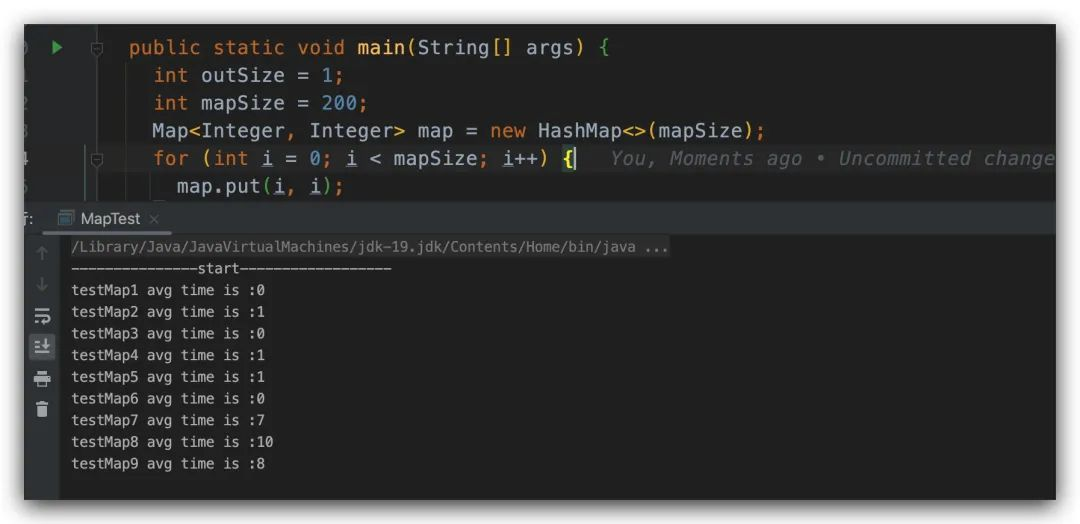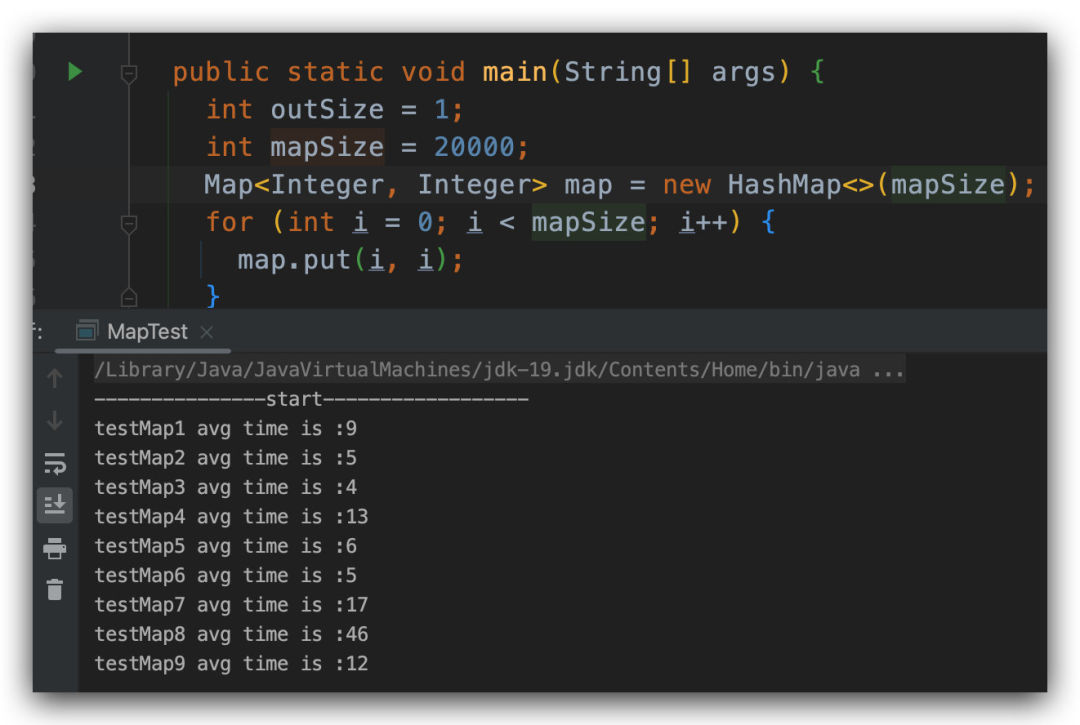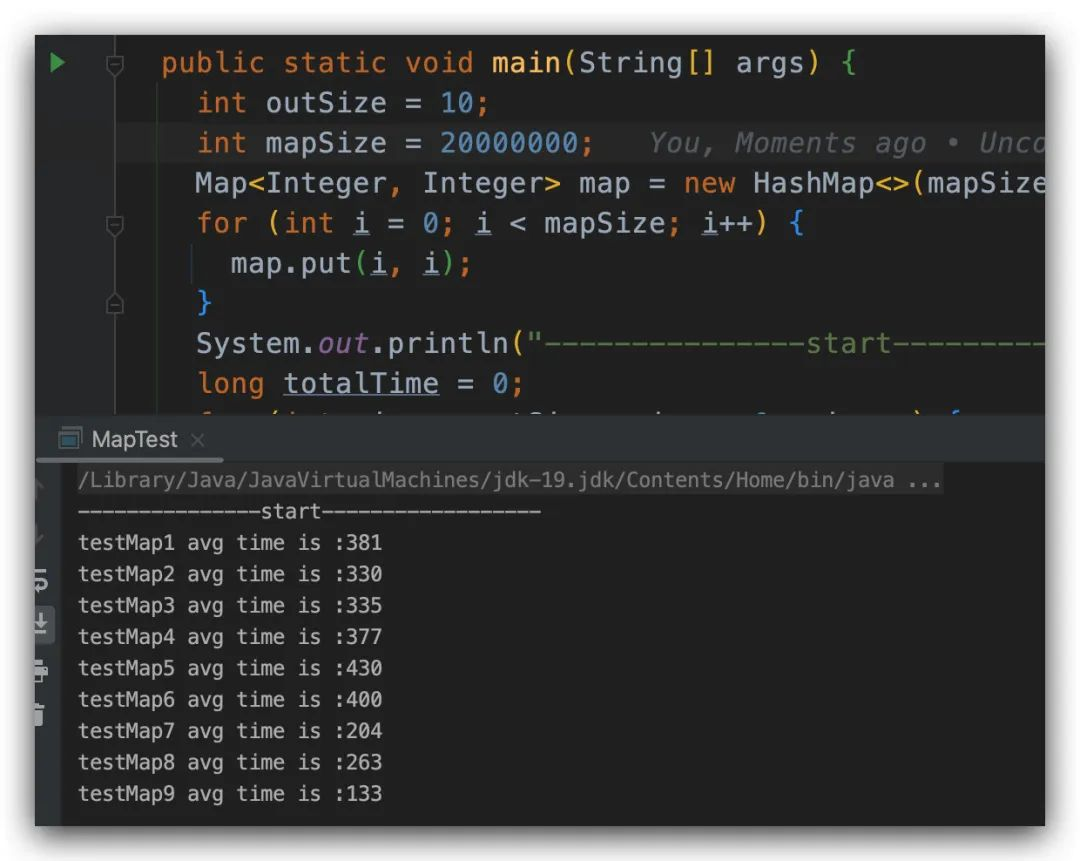0